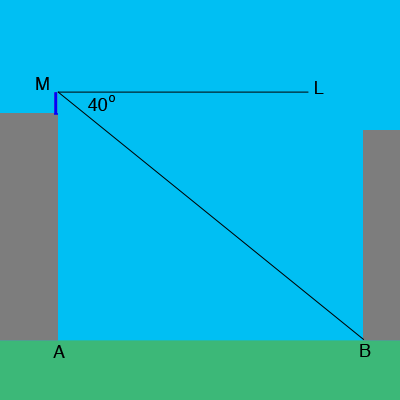SEARCH HOMEMath Central Quandaries & QueriesQuestion from kiki, a student: a man of height 1.5m standing on top of a building of height 48.5m views another building across the square. he observe that the angle of depression of the bottom of the building is 40 degrees and the angle of depression of the top of the building is 5 degrees. Both buildings stand on the same level a) calculate the distance of the man from the base of the building across the square measured along the line of sightHi Kiki,

In my diagram $M$ is the man's eyes, $A$ is the base of the building, $B$ is the base of the building across the square and $ML$ is a horizontal line.Triangle $ABM$ is a right triangle, and the length $|MA|$ is $48.5 + 1.5 = 50$ meters. What is the measure of the angle $BAM?$ What trig function relates the lengths of the sides $MA$ and $BM$ and the measure of the angle $BMA?$

PennyMath Central is supported by the University of Regina and the Imperial Oil Foundation.